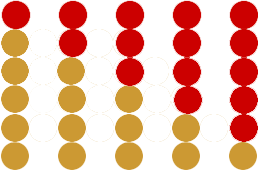Name: Stuart Who is asking: Other Level of the question: All Question: Hello there!! Please help, I'm stuck! Ok, so i am collecting 50p pieces and arranging them on my desk in the shape of a triangle. eg         50p      50p 50p   50p 50p 50p 50p 50p 50p 50p I want to work out how much money I'm saving just by knowing how many rows of coins there are. If i can work out how many coins there are just by knowing how many rows I have I can just divide by 2 to find out the amount in dollars. Cheers Stuart Stuart, If you have 1 row there is a total of 1 coin. If you have 2 rows there are a total of 3 coins. If you have 3 rows there are a total of 6 coins. If you have 4 rows there are a total of 10 coins. These numbers 1, 3, 6, 10,··· are called triangular numbers. Suppose you have 5 rows of 50p pieces.Slide the top 4 rows to the left to form a triangle with a right angle at the lower left corner.Now take the same number of 50p pieces, form the same triangle, rotate it 180 degrees and place it above your original triangle.What you have now is a rectangular array of coins with 6 rows and 5 columns and hence 65 = 30 coins. Half of them are the coins you started with and hence you started with  65 /2 = 15, 50p pieces. The same construction works for any row. If you have a triangle of n rows of 50p pieces then you have a total of  (n+1)n /2 50p pieces. Penny